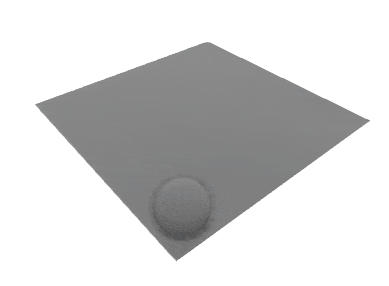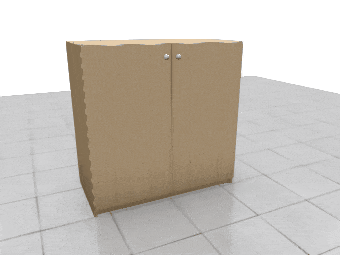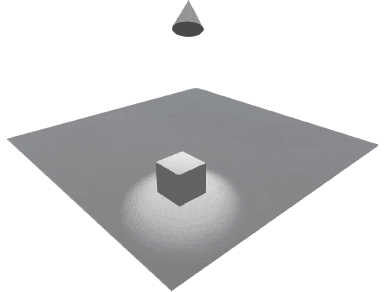# DriveWorks Pro 21How To: Understanding Vector Functions (KB20021401)

Send Feedback

## How To: Understanding Vector Functions

The inclusion of vector functions, in DriveWorks, is primarily intended to provide more freedom when it comes to controlling the position, rotation and scale of nodes in 3D documents.

Definition:

A vector is an object that has both a magnitude and a direction.

Geometrically, we can picture a vector as a directed line segment, whose length is the magnitude of the vector and with an arrow indicating the direction.

The direction of the vector is from its tail to its head.Frank D and Nykamp DQ

"An introduction to vectors."

From Math Insight.

The following Vector functions are available from the Rule Builder in DriveWorks:

## EulerCombine

VectorAdd can be used to combine positions, and VectorMultiply can be used to combine scale.

EulerCombine rotates the first given vector by the second, allowing rotations to be combined.

## VectorAbs

The absolute value of a number is how far away from zero it is, meaning it will always be nonnegative.

VectorAbs creates a version of the given vector using the absolute value of its components.

For example:

The absolute value of "7|-4|0" would be "7|4|0".

### Use Case

VectorAbs can be used to get the length of the specific axis of a vector.

For example:

The following rule would return the Z-axis length of the vector "-6|9|-11":

ListGetItem(VectorAbs("-6|9|-11"),3) will result in 11

## VectorAdd, VectorCross, VectorDot, VectorScale, VectorSubtract

Math Insight have an excellent introduction to vectors, and there are some great explanations and examples of these five functions written by Unity Technologies.

### Use Case

Smooth Translate accepts a pipe-delimited position as its Target. VectorAdd and VectorSubtract could be used to apply an offset to the entity's current target.VectorScale could be used to create an exploded view effect, where node positions scale from a center point using sliders.

VectorDot could be used to easily determine whether a clicked surface is considered flat (using the normal information provided by Touch Point Hit Position).

VectorCross can be used in conjunction with VectorSubtract to calculate the surface normal of a plane defined by a user (see Computing a Normal/Perpendicular vector for more information).

## VectorAngle

VectorAngle calculates the angle in degrees between two vectors.

For example:

The angle between "0|1|0" and "1|0|0" is 90°.

### Use Case

While rotating a node attached to a hinge using the Rotate entity, VectorAngle could be used to display the current angle, of the hinge mechanism, in degrees.## VectorClamp, VectorMax, VectorMin

VectorMin, VectorMax, and VectorClamp allow you to set minimum and/or maximum values for the components of a vector.

For example:

Using VectorClamp on the vector "-6|8|2", with "-4|-4|-4" as clamp vector 1 and "4|4|4" as clamp vector 2, results in "-4|4|2".

### Use Case

If a user is able to set the Target of a node's Animate Translation entity, VectorClamp could be used to sanitize the user input and prevent the node from traveling out-of-bounds.

## VectorDivide, VectorMultiply

VectorMultiply performs the pointwise multiplication of two vectors (x.x, y.y, z.z).

For example:

"2|7|-9" multiplied by "0|3|5" results in "0|21|-45".

VectorDivide performs the pointwise division of two vectors (x/x, y/y, z/z).

For example:

"-9|18|14" divided by "3|6|-7" results in "-3|3|-2".

### Use Case

VectorMultiply could be used to flip the value of the specific axis of a vector.

For example:

In order to flip "3|4|8" on the Y-axis, it can be multiplied by "1|-1|1", resulting in "3|-4|8".

## VectorLength, VectorNormalize

A vector has both magnitude and direction, and these two functions are used to obtain these properties individually.

VectorLength gets the length of a vector (losing directional information).

For example:

The length of "0|-6|8" is 10.

VectorNormalize gets a unit vector in the same direction as the vector (losing magnitude information).

For example:

"0|-6|8" normalized is "0|-0.6|0.8".

### Use Case

VectorNormalize can be used in conjunction with VectorScale to set the length of a vector, by first normalizing the vector and then scaling it (the scaling factor used will be the length of the new vector).

## VectorLerp

Lerp (a contraction of linear interpolation) is the simplest method of interpolation, and involves finding a value that is some percentage between two given values.

VectorLerp finds the vector that is a given percentage (value between 0 and 1) between two given vectors.

For example:

Linearly interpolating between "-5|-2|0" and "3|4|8" with an interpolation weighting amount of 0.5 results in "-1|1|4".

### Use Case

The value of a Slider Control could be used as the interpolation weighting amount used by VectorLerp in the rule for the position of a Node, allowing a user to control the position of the node between two points.

## VectorLookAt

The Get LookAt Rotation task and LookAt Entity can be used to calculate the required rotation for a node to face a target.

VectorLookAt differs in that it does not use node addresses for either of its inputs, solely using pipe-delimited positions.### Use Case

Instead of using the LookAt entity to make a node snap to face a target, VectorLookAt could be used in the Smooth Rotate Target property to make the node smoothly rotate to face a target.

## VectorNegate

The result of using VectorNegate is a vector with the same length but the opposite direction.

For example:

Using VectorNegate on the vector "0|-6|8" would produce "0|6|-8".

The length of both of these vectors is 10, but they are facing in opposite directions.

### Use Case

VectorNegate could be used to invert the Target of a node's Animate Translation entity, causing it to travel in the opposite direction, away from its original target.

## VectorRotateAroundAxis, VectorRotateAroundPoint

VectorRotateAroundAxis allows for a position vector to be rotated around an axis (defined by two position vectors) by a given number of degrees.

VectorRotateAroundPoint allows for a position vector to be rotated around another position vector by a given XY rotation.

### Use Case

VectorRotateAroundAxis could be used to create a complex hinge mechanism, without the need for a complex node structure.

Knowledge Base Article Ref: KB20021401